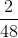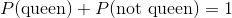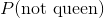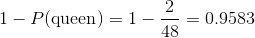Scan QR code or get instant email to install app

Question:

# From a pack of 52 cards which includes 4 kings, 4 queens, and 4 jacks. A king, a jack, a queen, and another queen are pulled without replacement. What is the probability of not selecting a queen on the next pull?

A 0.9583
explanation

1212Calculate the probability of selecting a queen from a deck that is missing a king, a jack, and two queens. Because 4 cards have been removed from the deck, and now there are only 2 queens remaining, the probability of selecting a queen is the ratio of the number of queens remaining, 2, to the total number of cards remaining, 48, or. However, the question wants the probability of NOT selecting a queen on the next draw. Recall that the probability of selecting a queen and the probability of not selecting a queen must sum to 1:. Rearrange to solve for: